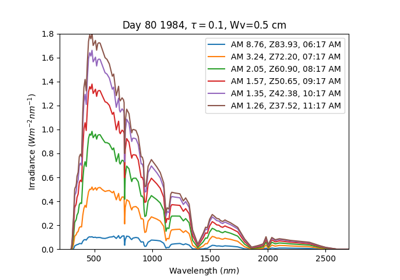Calculates the angle of incidence of the solar vector on a surface. This is the angle between the solar vector and the surface normal.

Input all angles in degrees.

Parameters
• surface_tilt (numeric) – Panel tilt from horizontal.

• surface_azimuth (numeric) – Panel azimuth from north.

• solar_zenith (numeric) – Solar zenith angle.

• solar_azimuth (numeric) – Solar azimuth angle.

Returns

aoi (numeric) – Angle of incidence in degrees.

## Examples using `pvlib.irradiance.aoi`#